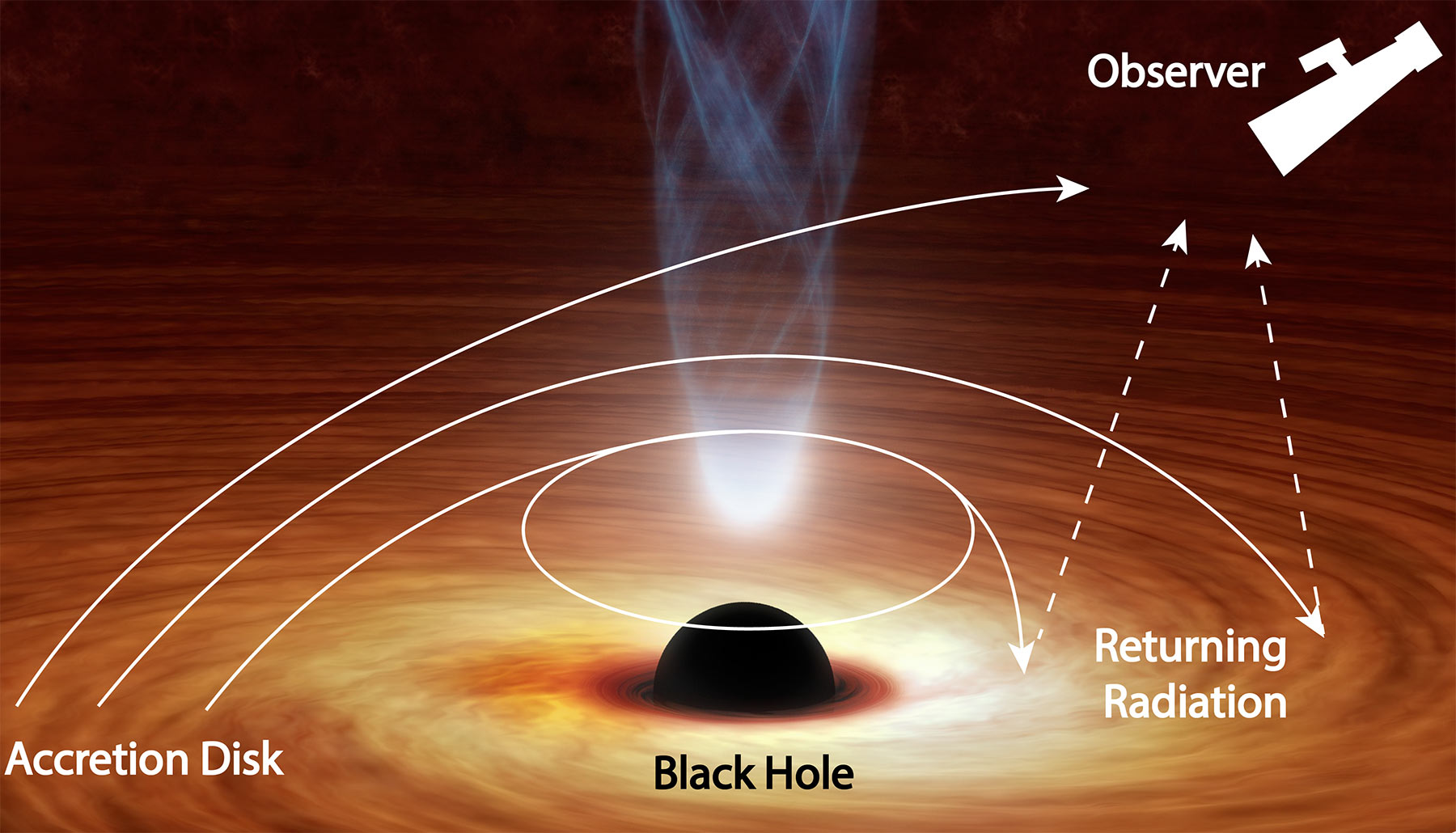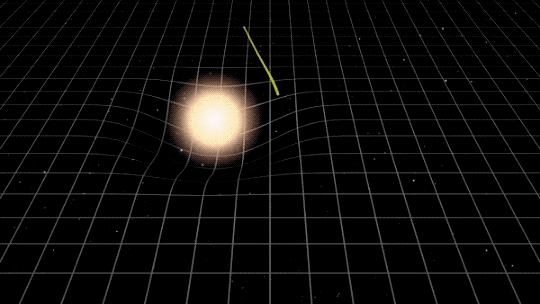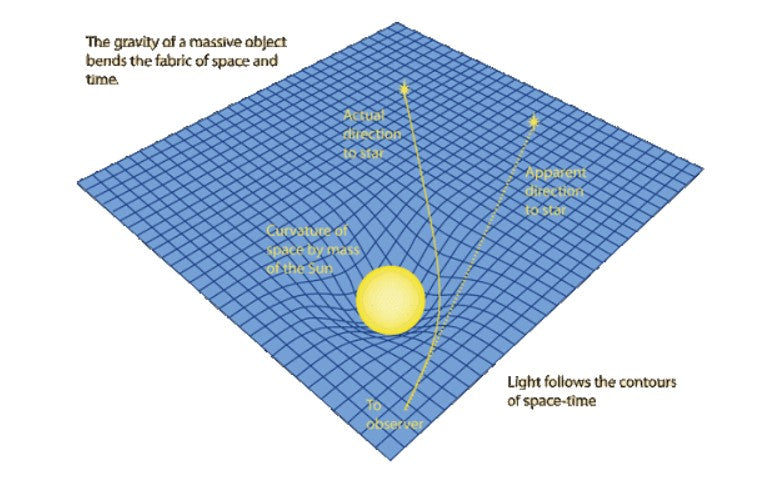# If Light Has No Mass, Why Is It Affected By Gravity?

It is that time of the week and we have selected an interesting question from a faithful follower involving the nature of space, mass and the magical nature of light.

"Hi ARSE, I was wondering why light is affected by gravity even though it has no mass? Is there more to the story?" - Adam, 53

Hi Andrew and thank you for inquiring about the nature of the universe.

This is a wonderful question. However, let's clarify a couple of things first.

1. Light bends by the effect of gravity; that is a fact.
2. Light has no mass ; thanks to this it can travel at the speed at which it does.
3. Light travels in a straight line.

So why does it curve?

Newton initially proposed that gravitational attraction between two bodies is inversely proportional to the square of the distance between them and proportional to the product of their masses. In other words, dough attracts dough on a cutting board. To this day, we thank Newton for giving us a formula for calculating this attraction.

Then in 1915, a great physicist by the name of Albert Einstein proposed the "Theory of General Relativity". He suggested that gravity is not an attractive force between two bodies, but rather the deformation of a tissue that he called spacetime.

I'll explain it to you with the classic example: If you have a mesh, and you place a marble in it, the mesh will curve, causing any body in it to move towards the marble you placed in the first place. Thus, the Sun, for example, warps space-time causing the trajectories of everything around it (such as the Earth) to be affected.

“But light travels in a straight line ... right???”Yes. It does.

But the space-time in which it travels is curved (exerting gravity). Thus, it seems that light is bent by the effect of this gravity. Or rather, it does; It curves! It is not a matter of perspectives, it is a fact. But in reality, it is travelling in a straight line through the continuous fabric of space-time, which, by the presence of energy (mass of another body), bends.

I attach the following illustration to complement the explanation:QUESTION: Did you know any of this? At all?
Let us know and we’ll post more questions and answers.

Keep thrusting Australia into the deep unknown…

#Space_Aus